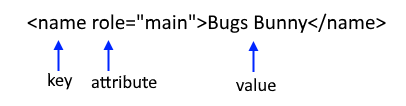FreeKB - Perl (Scripting) - XML::Simple printing keys (XMLin)
Perl (Scripting) - XML::Simple printing keys (XMLin)Updated:   |  Perl (Scripting) articles

Let's consider this XML.

``````<acme>
<name role="main">Bugs Bunny</name>
</acme>``````Data::Dumper can be used to understand how XML::Simple is interpreting the XML. Here is an example of how to use Data::Dumper.

``````#!/usr/bin/perl
use strict;
use warnings;
use XML::Simple;
use Data::Dumper;

my \$xml= XMLin("example.xml");
print Dumper \$xml;``````

Which will produce the following.

• "name" is the root key
• "content" and "id" are child keys below the root key
• "Bugs Bunny" is a value of the "content" key
• "1" is a value of the "id" key
• Data inside of { } is interepreted as a hash
``````\$VAR1 = {
'name' => {
'content' => 'Bugs Bunny',
'role' => 'main'
}
};``````

To loop through each root key:

``````foreach my \$root_key (keys %{\$xml}) {
print "\$root_key\n";
}``````

Which, in this example, will print "name".

``name``

To loop through each child key.

``````foreach my \$root_key (keys %{\$xml}) {
print "root key = \$root_key\n";
foreach my \$child_key (keys %{\$xml->{\$root_key}}) {
print "child key = \$child_key\n";
}
}``````

Which, in this example, will print the following.

``````root key  = name
child key = content
child key = role``````

Or, if you already know the child key, you could do this.

``````foreach my \$child_key (keys %{\$xml->{'name'}}) {
print "child key = \$child_key\n";
}``````

If so, consider buying me a coffee over at